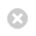Close

UCL Great Ormond Street Institute of Child Health

HomeGreat Ormond Street Institute of Child Health# Section 3: Power

The power of a study is its ability to detect a difference of a given size, given that the difference actually exists. For a fixed sample size, larger differences can be detected with greater power. Excel sheets are given for the calculation of sample sizes to detect specified differences with chosen power and significance; these are known as power calculations.

For each sample size calculation, the size of difference, d, to be detected should be given. 'd' is the minimum difference deemed to be of clinical importance.

In each excel sheet (except time to event) there is a section that gives the required sample size for a specified power, significance and difference. In some sheets there is also a lower section that gives the power of a study to detect a specified difference at a chosen significance level with a set sample size. The latter sections may be useful where numbers are restricted and the power available is useful to know.

[3.1] Means

Below are links to excel spreadsheet (.xls) for calculating sample size for a single mean or for the difference between two means using the power approach to sample size estimation.

## Power - one meanPower - one mean

## Power - difference between two meansPower - difference between two means
[3.2] Proportions and Percentages

Below are links to excel spreadsheet (.xls) for calculating sample size for a single proportion or percentage or for the difference between two proportions or percentages using the power approach to sample size estimation.

## Power - one proportionPower - one proportion

## Power - difference between two proportionsPower - difference between two proportions

## Power - one percentagePower - one percentage

## Power difference between two percentagesPower difference between two percentages
[3.3] Rates

Below are links to excel spreadsheet (.xls) for calculating sample size for a single rate or for the difference between two rates using the power approach to sample size estimation.

## Power - one ratePower - one rate

## Power difference between two ratesPower difference between two rates
[3.4] Time to Event

Below is a link to an excel spreadsheet (.xls) for calculating sample size for a time-to-event outcome using the power approach to sample size estimation.

## Power - time to eventPower - time to event